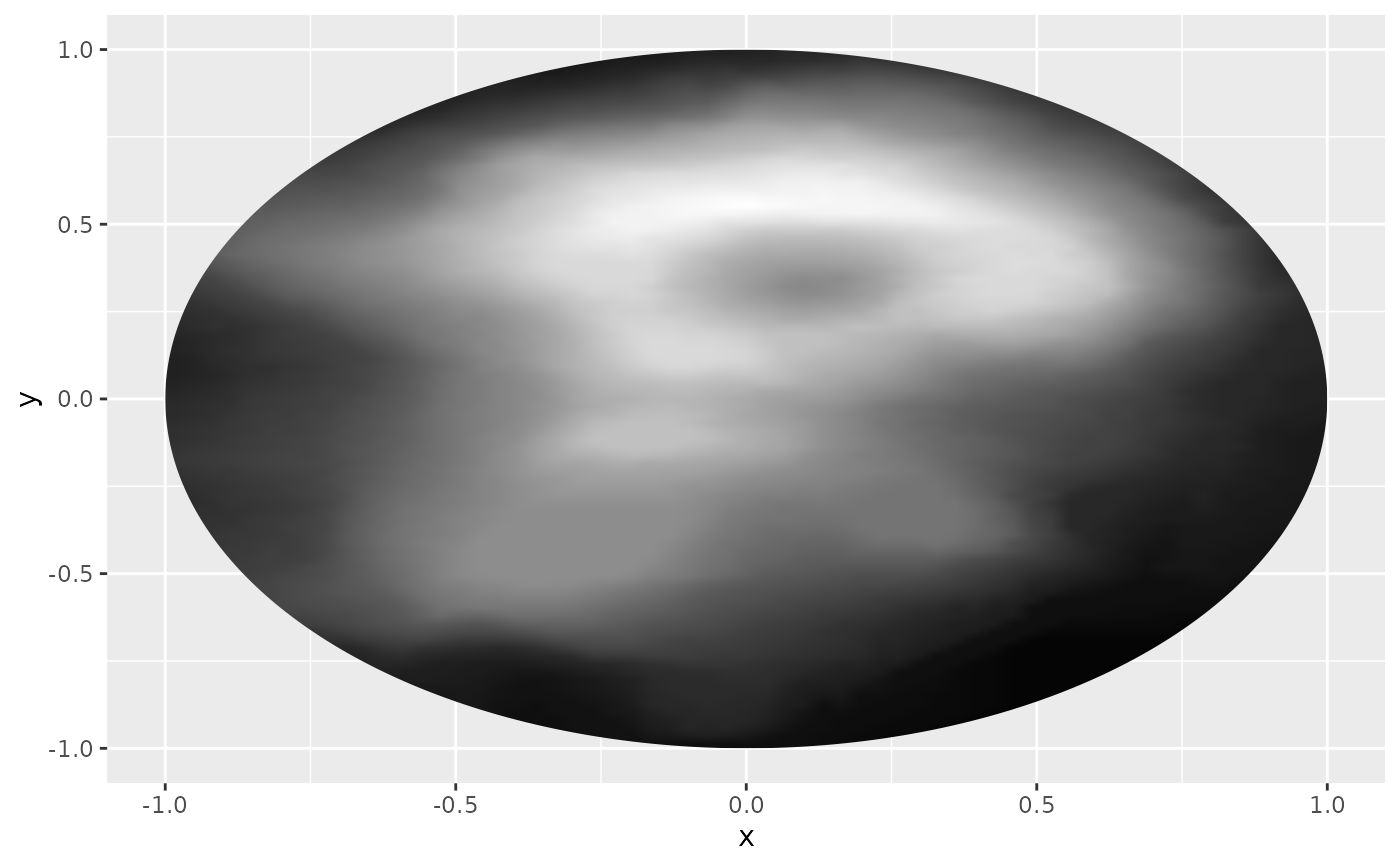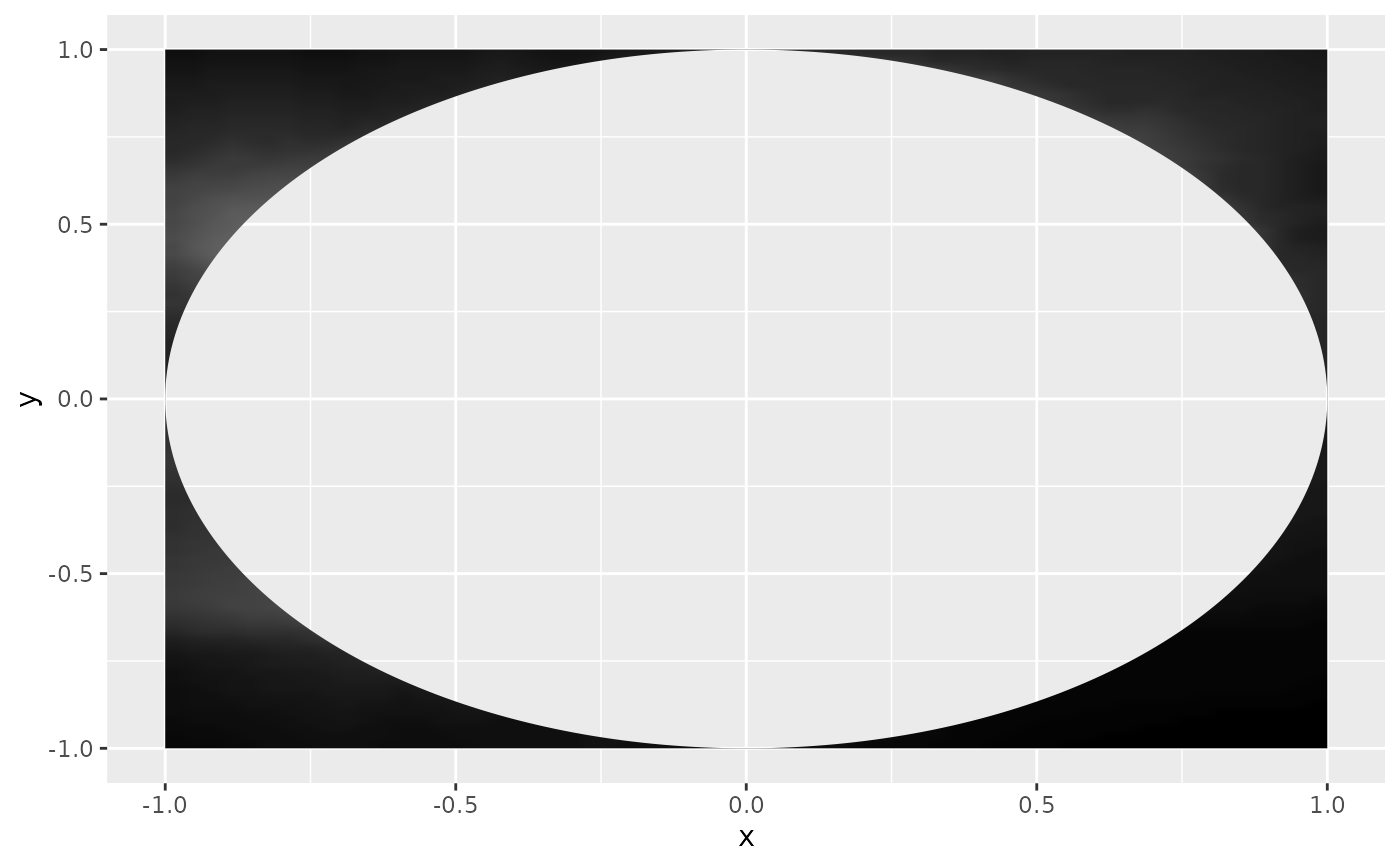This filter applies a mask to the given layer, i.e. sets the opacity of the layer based on another layer

with_mask(x, mask, invert = FALSE, ...)

## Arguments

x

A ggplot2 layer object, a ggplot, a grob, or a character string naming a filter

The layer to use as mask. Can either be a string identifying a registered filter, or a raster object. Will by default extract the luminosity of the layer and use that as mask. To pick another channel use one of the channel specification function.

invert

Should the mask be inverted before applying it

...

Arguments to be passed on to methods. See the documentation of supported object for a description of object specific arguments.

## Value

Depending on the input, either a grob, Layer, list of Layers, guide, or element object. Assume the output can be used in the same context as the input.

Other blend filters: with_blend_custom(), with_blend(), with_interpolate()

## Examples

library(ggplot2)
volcano_raster <- as.raster((volcano - min(volcano))/diff(range(volcano)))
circle <- data.frame(
x = cos(seq(0, 2*pi, length.out = 360)),
y = sin(seq(0, 2*pi, length.out = 360))
)

ggplot() +
as_reference(
geom_polygon(aes(x = x, y = y), circle),
id = 'circle'
) +
annotation_raster(volcano_raster, -1, 1, -1, 1, TRUE),
)# use invert = TRUE to flip the mask
ggplot() +
as_reference(
geom_polygon(aes(x = x, y = y), circle),
id = 'circle'
) +